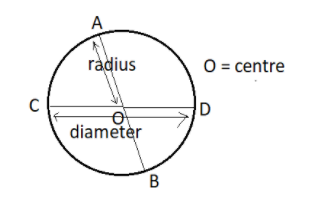QuestionAnswers

# Fill in the blanks with correct word(S) to make the statement true:Radius of a circle is one half of its ____________ .Verified
128.4k+ views
Hint: The Diameter is double the radius in a circle. Radius in a circle Is the distance from the centre of the circle to its boundary.Now, in a circle the radius is the length or the distance from the centre to the boundary of the circle. From the figure radii are $OA,OB,OC and OD$. We know that all radii in a circle are of equal length. So then $OA = OB = OC = OD$ .
The lines drawn in a circle, passing through the centre of the circle, joining two points across the boundaries are all diameters. For the circle given in the figure the diameters are AB and CD and we can see that $AB = AO + OB$.
$AB = AO + AO \\ \Rightarrow AB = 2AO \\ Or,\dfrac{{AB}}{2} = AO \\ \Rightarrow AO = \dfrac{{AB}}{2} \\$